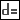Relations (also known as parametric relations) are user-defined equations written between symbolic dimensions and parameters. Relations let you capture design intent by defining relationships within features or parts, or among assembly components.
You can use relations to control the modeling process in the following ways:
To control the effects of modifications on models
To define values for dimensions in parts and assemblies
To set constraints for design conditions. For example, by specifying the location of a hole in relation to the edge of a part.
To describe conditional relationships between different parts of a model or assembly
Relations can be simple values (for example, d1=4) or complex, conditional, branching statements.
You can also use units in relations. For more information on units, click here.
Accessing Relations
To access relations, open a model and click Tools >Relations. The Relations dialog box opens.
Relation Types
There are two types of relations:
Equality—Equates a parameter on the left side of the equation with an expression on the right side. This type of relation is used for assigning values to dimensions and parameters. For example:
Simple assignment: d1 = 4.75
More complex assignment:
d5 = d2*(SQRT(d7/3.0+d4))
Comparison—Compares an expression on the left side of the equation with an expression on the right side. This type of relation is commonly used as a constraint or in conditional statements for logical branching. For example:
As a constraint:(d1 + d2) > (d3 + 2.5)
In a conditional statement: IF (d1 + 2.5) >= d7